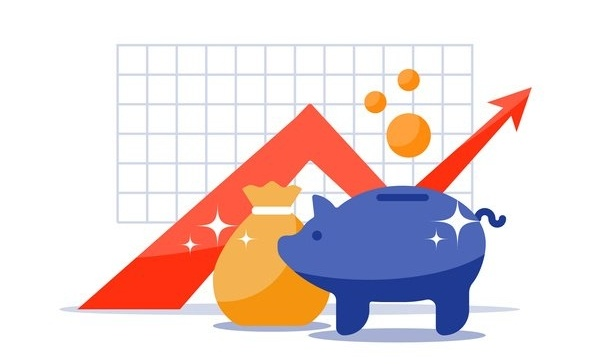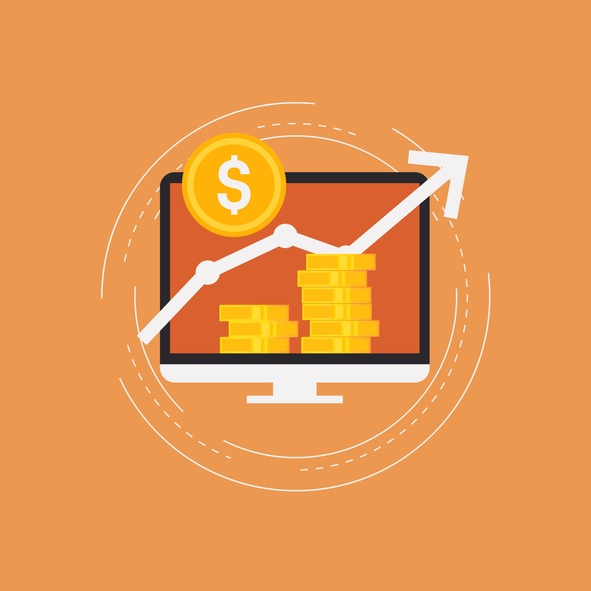# DeFreitas & Minsky Accounting BlogIt’s something that is part of our daily lives. It determines how some of your investments grow, and how much money you may owe a lender. But, do you actually know what is compound interest? It is an important part of financial literacy, which is surprisingly simple to learn.

## What is Compound Interest?

Compound interest is a type of interest that is calculated on the initial principal and also includes all of the accumulated interest of previous periods. Not all types of interest compound in the same way. They accrue at a rate that depends on the frequency of compounding. The more compounding periods there are, the greater the compound interest will ultimately be.

## What is Compounding Interest used for?

Compounding is used in many common interest calculations, like savings accounts and loans. It is also used in stocks that pay investors dividends. If you are interested in growing your nest egg, compound is usually more beneficial to you.## How it is Calculated

To calculate compound interest, multiply the initial principal amount by one plus the annual interest rate raised to the number of compounding period minus one. Then, subtract the total initial amount of the principal from the resulting value.

= [P (1 + i)n] – P

For example, a three-year, \$100.000 investment, which has 5 percent compounding interest, would be calculated like this:

\$100,000 [(1+0.05)3-1] = \$100,000 [1.157625-1] = \$15,762.50.

## Frequency can make a Big Difference

Compound interest can be calculated based on any frequency schedule, including daily, monthly or hourly. However, savings banks usually compound interest based on a daily schedule. If the interest is calculated more frequently, it works out to the benefit of investors or creditors. Less frequent periods are more beneficial to borrowers.## Simple vs. Compound Interest

Although compound interest increases annually, it does not grow at the same rate each year. It takes into consideration the accumulated interest of the previous periods.

However, simple interest is measured in slightly different way. It means that the amount of money accrued is based on a set percentage, which never changes.

Simple interest is calculated by multiplying the daily interest rate by the principal of the loan by the number of days between payments. It is typically used in automobile loans, short-term loans and some types of mortgages.

If you still have questions — like “what is compound interest?” or “how can I best take advantage of compounding?” — reach out to a local tax professional who can discuss personal financial planning options with you.Topics: Financial Planning

## DM CPA Firm Blog

### Find answers to any accounting questions you have.

These questions are usually from our clients.  If you would like to send us a question, please email us at info@dmaccountingfirm.com or ask on one of our social accounts.